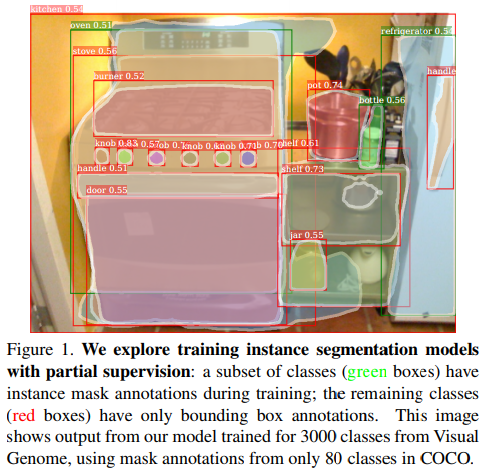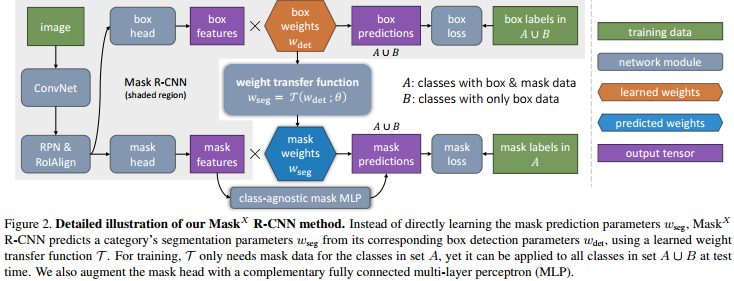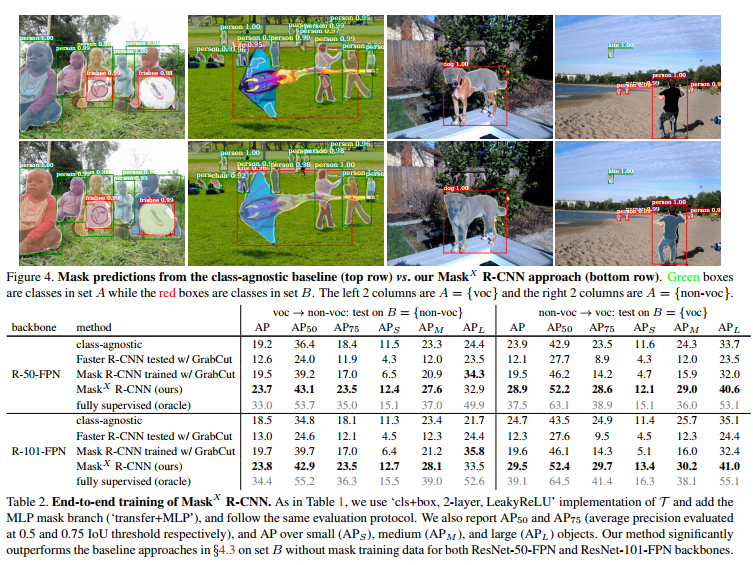### Description

Most methods for object instance segmentation require all training examples to be labeled with segmentation masks. This requirement makes it expensive to annotate new categories and has restricted instance segmentation models to well-annotated classes. The goal of this paper is to propose a new partially supervised training paradigm, together with a novel weight transfer function, that enables training instance segmentation models on a large set of categories all of which have box annotations, but only a small fraction of which have mask annotations. These contributions allow to train Mask R-CNN to detect and segment visual concepts using box annotations and mask annotations.### Proposed method

The idea is that instead of learning the Mask R-CNN bounding box head and mask head independently, they use the bounding box head to predict the mask head parameters using a generic, category-agnostic weight transfer function that can be jointly trained as part of the whole model.

In other words, instead of learning the segmentation weights $$w^c_{seg}$$ directly as usual, they learn it via a transfer function $T(.)$ :

$w^c_{seg} = T(w^c_{det},\theta)$

where $$w^c_{det}$$ are the bounding box head weights. In their implementation, $$T(.)$$ is a fully connected neural net. C.f. Fig.2 for more details.### Results

They get good results, at least better than by training Mask R-CNN with GrabCut.